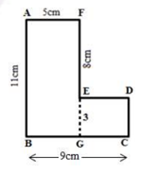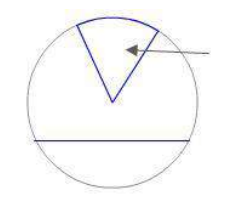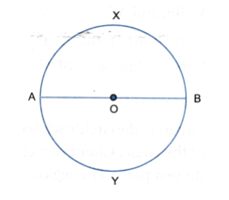# ICSE Class 6 Maths Important Questions

The Indian Certificate of Secondary Education is an examination conducted by the Council for the Indian School Certificate Examination. The ICSE is responsible for framing the curriculum and designating the topic for Class 6 students. Class 6 Maths important questions has been created with a whole goal to help students quickly learn mathematical concepts and develop higher logical reasoning and problem-solving skills. ICSE Class 6 Maths Important Questions are prepared by subject experts following the ICSE Class 6 Maths Syllabus prescribed by the Council.

Going through these important questions of Maths along with other reference study materials will help students to prepare themselves effectively. Once they complete the entire syllabus they should practice these important questions to make their concepts more clear. Students of ICSE Class 6 can go through the important questions of maths provided below and should practice it on a regular basis.

Students of Class 6 can practise the ICSE Class 6 Important Questions of Mathematics provided below to revise the entire syllabus in a quick span of time.

1. Find the sum of the following: -146, -78, 124, 69
2. Use the divisibility tests to determine whether the number 378 is divisible by 2, 3, 4, 5, 6, 9 and 11.
3. The monthly income of Arun and Varun are Rs. 18000 and Rs. 27000 respectively. What is the ratio of the income of Varun to that of Arun in its simplest form?
4. Ananya along with her 2 friends ordered one sandwich each at their favourite restaurant. They left a tip of 7 rupees for the waiter. If they spent a total of a hundred rupees, find the cost of each sandwich. Frame an equation for the given situation and then solve the same.
5. Let A = {x: x is a letter in the word CHANDIGARH} and B = {x: x is a letter in the word RAJASTHAN}
1. Find A ∩ B and A ∪ B
2. Find n(A), n(B), n(A ∩ B) and n(A ∪ B)
3. Verify: n(A ∪ B) = n(A) + n(B) – n(A ∩ B)
6. If the area of a rectangular plot is 144 sq. m. and its length is 16 m, find the breadth of the plot. Also, find the cost of painting a blue border around the plot if the cost is Rs. 3 per meter.
7. The table represents the number of books sold in a store during four months of a year. Make a bar graph to represent the data.
 Months January February March April No. of books 140 120 110 130
8. In the given figure, all the adjacent sides are at right angles.Find:
1. The perimeter of figure
2. Area of the figure.9. A painter can paint a wall of area 20 in 10 hours. If he works at a constant speed, how much time will he take to paint a wall of area 30?
10. If the H.C.F. of two numbers is 24 and their product is 5760, find their L.C.M.
11. Write the following numbers in the descending order of their values and represent them on a number line.
1. 8, -6, 2, -12, 0, 3, 15 and -1
2. Integers greater than -6 and less than 2.
12. Express each of the following as an algebraic expression.
1. Sum of y and 7.
2. Number m divided by 23 and added to 5
13. Name the following:
1. Name the two parts of the circle in blue.1. What is AB and how does it divide the circle.14. A vegetable trader buys some tomatoes and onions for Rs. 420 such that the ratio of the total weight of tomatoes to the total weight of onions is 2:3. The total weight of the tomatoes and onions is 60 kg. If the ratio of the total price of tomatoes to the total price of onions is 8:27, then what is the cost of 5 kg of tomatoes and 5 kg of onions?
15. Find the value of: 34+5, where
16. Name the types of the following sets:
1. Set of even numbers which are not divisible by 2.
2. {Number of people in India}
3. Set of odd numbers between 7 and 19.
17. Write each of the following sets in roster form as well as in set builder form:
1. Set of factors of 48
2. Set of integers between -3 and 8
18. What is the central angle of the sector corresponding to the expenditure incurred on Royalty?
19. State whether true or false:
1. {3, 5, 7…} is a finite set.
2. A line has infinite number of points on it.
3. 0.45 = 45%
20. The sides of a triangle are in the ratio 3:2:4. If the perimeter of the triangle is 27cm, find the length of each side

### Advantages of solving ICSE Class 6 Maths Important Questions

• It helps them to revise the important numerical problems from each chapter.
• The important questions of Maths cover the entire Class 6 Maths syllabus.
• Practising these important questions will help students in boosting their confidence level.
• It will help them evaluate their performance level and work on their weak points.